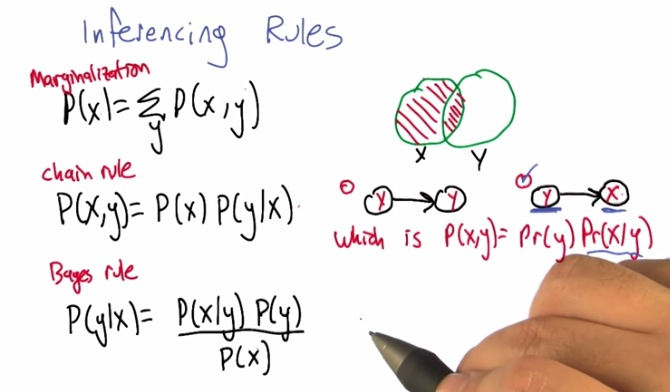• Marginalization: to put X, simply count what's not y, and what's y and x, that will produce x
• Chain Rule: don't cross the x dependent on y, because that only tell us that that p(x) is independent of y(where it's actually the case where both x and y occurs)
• And the usual, Bayes' Rule, altogether with the three makes all probability for any given arguments.
• On the problems above, the the formula should be represented by the second graph. Because as we talked earlier, the probability of y should be known, and thus y is the origin, which makes the probability of x is inference. Although it's the arrow, it's x and y is conditional independence.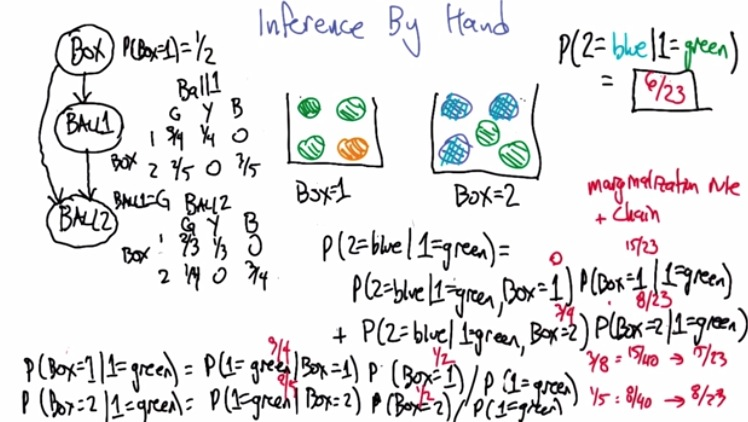• Le't put altogether those 3 rules to put into action.
• Suppose there's two box, each filled of balls.
• What's the probability that we get ball in a bax green, and green, in order? (The ball is not return to the bag)
• Well, there's two box.  But the one that filled with both blue and green is only box 2. So we don't need to take box 2 into account. So this way, we don't need to take all the trouble to calculate the probability of box 1.
• So in any of this case, we choose(optionally) the marginalization rule.
• First we take into account the probability we pick ball green from each of the box.
• Well, that means, we apply Bayes' Rule. But as we apply Bayes' Rule, we need to kow the probability of ball picked green, to normalize the term. Turns out we don't need too. We just take the other case(where we picked the green from the box). So, we take first ball green from box 1 and first ball green from box 2 is complementary. That both will equal itself the probability we pick first ball green. In other words, we just take both probability and normalize both of them.  so 3/4 P(1=G|Box=1)xP(Box=1) equals 3/8, P(1=G|Box=2)xP(Box=2) equals 1/5. Normalize both of them give 15/23 and 8/23 each. Now since we only need box 2, we picked the second ball in box 2, which previously picked first ball=green, give us 3/4. So 3/4x8/23 = 6/23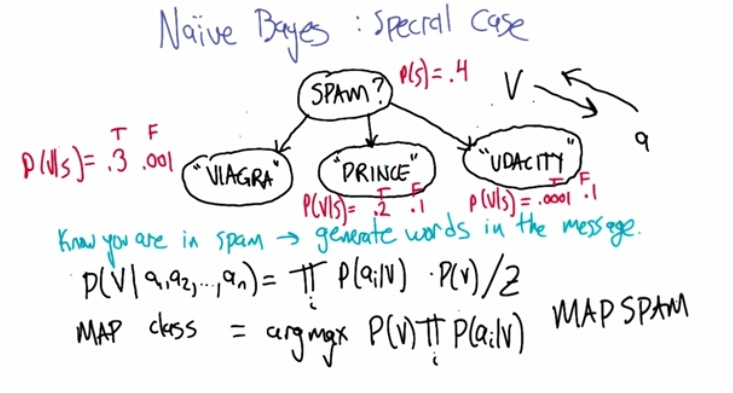• Now here we can take spam classification example as Bayes Learning Problem.
• Let's say that one's email folder has 40% email, or 0.4.
• And in spam, there's also many type of spam, viagra, prince, udacity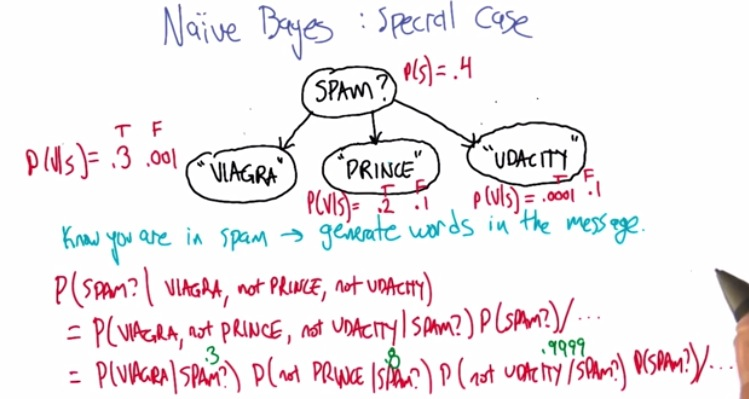• Now, here's some general question machine learning problem. What is the probability of the email is spam considering the email has 'viagra', 'not prince', and 'not udacity'?
• As we know that prince'udacity'viagra is conditionally independence each other, we can take separate question. So we can takeout each probability separately. We don't have to calculate dependance of all another. Just take each of the probability given the condition and we're good to go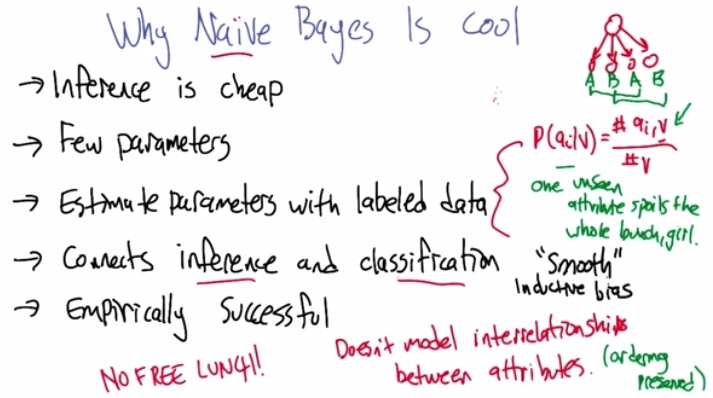• The inference is cheap. We know that most of usual learning algortihm. it will much harder, the cost is np samples given parameters.
• But with this, we only have some linear examples. Because Bayes only concern(naively) about one parameters impact to the label.
• It only has few parameters.
• Estimate parameters with labeled data.We can estimate the value of parameters. How we do that? By probability, we can tell the exact probability given labeled class, by counting how much atrributes in that labeled class, across all labeled class, and thus we have value for particular attribute given the class.
• Connect inference and classfication. This is incredible as we can connect inference to machine learning problems, for example, classfication.
• Empirically successful. Many people, including Google Giant, use Bayes Naive extensively in all of other problems.
• So why do we even need other algorithms? We only need this algorithm should we?  Is it no free lunch?
• The other downside maybe, is we don't consider, whether each attributes have particular relationship over the other.
• Suppose we have the first attribute correlated with third attribute. and fourth attribute correlated with second attribute. Well with given formula above, we only end up putting more weight for attribute (2 for 1) and we can avoid the rist overfitting.
• The other thing is when we have every other attributes said yes (classfication label) but the label, since the value(v=0), thus a1xa2x...0= 0. So we  get no. This could be dangerous, as if we haven't seen one attribute in one sample, and it just bluntly said no.
• Well what we could, is smooth the 'unseen parameter' with value more than zero. But be careful as we do random initilization we can risk about being overfitting.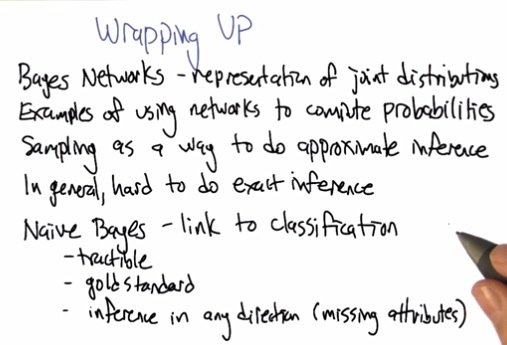• We talked about Bayes networks, and it's representation of join distribution.
• Using spesial Examples.
• Samping to do approximate inference (which also hard)
• And Naive Bayes is also the gold standard like in Bayesian Learning.
• Inference any direction(for labeled,also for missing attribute). In decision tree, we might get stuck if we have missing attribute for particular samples, but Bayes can infer what the missing attributes, by just looking this particular attribute value across all of the examples.